# Cross Spectrum Computation

#### 2020-06-23

Here I show how the modeling tools in kitagawa can be used to study actual data. Specifically, I will show records of strain and pore-pressure from borehole stations in the [Network of the Americas(https://www.unavco.org/projects/major-projects/nota/nota.html)1 in a manner similar to the approaches taken in Barbour (2015).

library(kitagawa)
## Loaded kitagawa (3.1.0) -- Spectral response of water wells; see ?kitagawa

## Pore Pressure Changes from Teleseismic Strains

We first load the psd package, which includes a suitable dataset for this example. In particular, we’re interested in assessing the frequency-dependent relationship between pore pressure $$p$$ and areal strain $$E_A$$2 during the seismic portion of the record.]

library(psd)
## Loaded psd (2.0.0) -- Adaptive multitaper spectrum estimation; to start, see ?pspectrum
data(Tohoku)
toh_orig <- with(subset(Tohoku, epoch=='seismic'), {
cbind(
scale(1e3*areal, scale=FALSE), # scale strain to nanostrain, remove mean
scale(1e2*pressure.pore, scale=FALSE) # scale hPa to Pa, remove mean
)
})
colnames(toh_orig) <- c('input','output')
toh.dat <- window(ts(toh_orig), 100, 2400)

Note how the records of this earthquake – the 2011 $$M_W 9$$ Tohoku-Oki earthquake some thousands of kilometers away – are very nearly a mirror image of each other: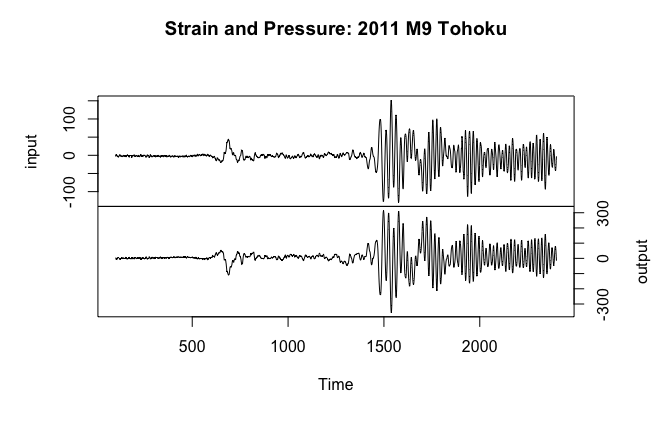The energy carried by the seismic wavetrain is focused predominately at long periods and for the surface waves it is very nearly harmonic. Zooming in on the early part of the Rayleigh wave: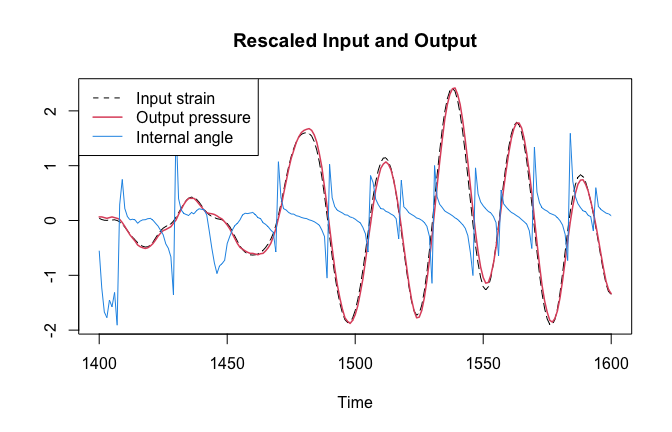we see a close mirroring of the input and the output, with the input occurring before the output. The tangent of the angle between the signals during the periods of more purely harmonic strain is suggestive of a simple phase lag. In other words, the pore pressure lags the strain. These observations are consistent with the theory of linear poroelasticity, which predicts the following response in undrained conditions (assuming an undrained Poisson’s ratio of $$1/3$$): $p \approx - \frac{4}{3} B \mu E_A$ where $$B$$ is the Skempton’s coefficient and $$\mu$$ is the elastic shear modulus of the fluid-saturated rock. All of this indicates that the pore pressure response can be modeled as a convolution of an input signal (dynamic strain) and transfer function ($$p = G \star E_A$$).

In this case the (scalar) proportionality implied by the timeseries is

m <- lm(output ~ input - 1, as.data.frame(toh.dat))
strain_scaling <- coef(m)
signif(strain_scaling, 3) # GPa/strain
## input
## -2.27

but we will see how this is actually frequency dependent.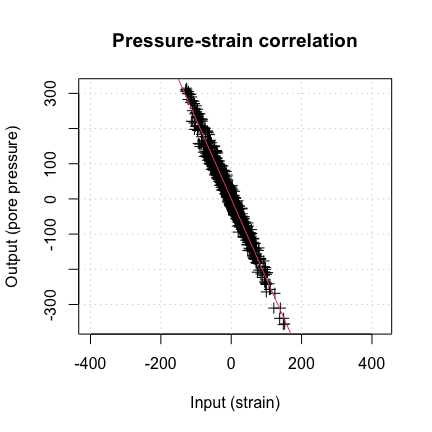## Cross-Spectrum Estimation

If the results of the spectral computation include the complex auto spectra and cross spectrum $$[S_{11}, S_{12}, S_{22}]$$, the coherence spectrum $$\gamma^2$$ can be calculated by $\gamma^2 = \frac{\left|S_{12}\right|^2}{S_{11} S_{22}},$ the admittance spectrum (or gain) $$G$$ can be calculated from $G = \gamma \sqrt{S_{22} / S_{11}},$ and the phase spectrum $$\Phi$$ can be calculated from $\Phi = \arg{S_{12}}$ As Priestley (1981) shows, the multitaper coherency spectrum ($$\gamma$$) can be described by an distribution: $\frac{2 k \gamma}{(1-\gamma)} \sim F(2,4k)$ Hence, the probability that the absolute coherency is greater than $$c$$ is $P(|\gamma| \geq c, k) = (1 - c^2)^{k-1}$

k <- 2*130 # number to start out with
gam <- seq(0.001, 1, by=0.001)
gamrat <- 2 * gam / (1 - gam)
Pgam <- pf(k*gamrat, 2, 4*k)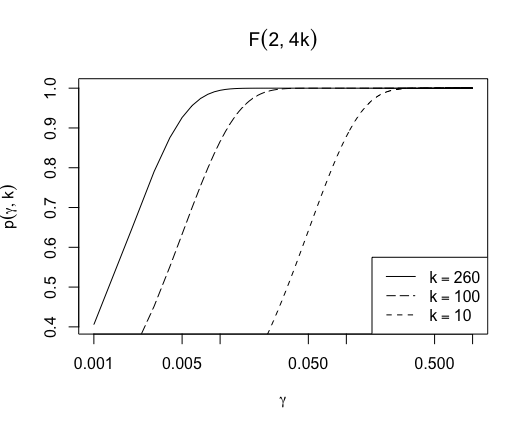The standard error in the admittance follows from the coherence spectrum: $\sqrt{(1 - \gamma^2)/k}$

## Application to Tohoku record

First let’s use psd to estimate a cross spectrum between pressure and strain, treating strain as the input to the system and pressure as the output.

#!order_matters
class(toh.dat)
##  "mts"    "ts"     "matrix"
toh_to_pspec <- toh.dat[,c('input','output')]
toh.cs <- psd::pspectrum(toh_to_pspec, ntap.init=k, verbose=FALSE)

Which gives the following results:

class(toh.cs)
##  "amt"  "spec"
str(toh.cs)
## List of 21
##  $freq : num [1:1150] 0 0.000435 0.00087 0.001305 0.001741 ... ##$ spec             : num [1:1150, 1:2] 56786 56767 56708 56609 56470 ...
##  $pspec : cplx [1:1150, 1:2, 1:2] 1.29e+08+0i 1.29e+08+0i 1.29e+08+0i ... ##$ transfer         : cplx [1:1150, 1] -2.47+0i -2.47-0.01i -2.47-0.02i ...
##  $coh : num [1:1150, 1] 0.894 0.894 0.894 0.894 0.894 ... ##$ phase            : num [1:1150, 1] -3.14 3.14 3.14 3.13 3.13 ...
##  $kernel : NULL ##$ df               : num 634
##  $numfreq : int 1150 ##$ bandwidth        : num 0.277
##  $n.used : int 2300 ##$ orig.n           : int 2301
##  $series : chr [1:1153] "structure(c(1.7458842897322, 3.7458842897322, 0.745884289732203, " "-5.2541157102678, -8.2541157102678, -5.2541157102678, -3.2541157102678, " "-5.2541157102678, -6.2541157102678, 1.7458842897322, 2.7458842897322, " "1.7458842897322, 3.7458842897322, 5.7458842897322, 5.7458842897322, " ... ##$ snames           : chr [1:2] "output" "input"
##  $method : chr "sine multitaper" ##$ taper            : 'tapers' int [1:1150] 93 93 93 93 93 93 93 93 93 94 ...
##   ..- attr(*, "last_recorded")= logi NA
##   ..- attr(*, "n_taper_limits_orig")= num [1:2] 1 317
##   ..- attr(*, "taper_positions")= logi NA
##   ..- attr(*, "span_was_set")= logi FALSE
##   ..- attr(*, "n_taper_limits")= int [1:2] 36 317
##  $pad : logi TRUE ##$ detrend          : logi FALSE
##  $demean : logi FALSE ##$ timebp           : num [1:1150] 46.5 46.5 46.5 46.5 46.5 46.5 46.5 46.5 46.5 47 ...
##  \$ nyquist.frequency: num 0.5
##  - attr(*, "class")= chr [1:2] "amt" "spec"

We form the requisite quantities, which are included as output from pspectrum:

f <- as.vector(toh.cs[['freq']]) # frequency
lf <- log10(f)
p <- 1/f # period

# coherence
Coh <- toh.cs[['coh']]
Phi <- as.vector(toh.cs[['phase']])
suppressPackageStartupMessages(can.unwrap <- require(signal))
if (can.unwrap){
# unwrap if possible
Phi <- signal::unwrap(Phi)
}
G <- Mod(toh.cs[['transfer']])
G <- Coh * G
# Tapers
K <- toh.cs[['taper']] # 'tapers' object
k <- as.numeric(K)
G.err <- sqrt((1 - Coh) / k)

We can safely assume that the spectral density estimates for periods longer than $$\approx 100$$ seconds will be either spurious, or lacking in seismic energy, so we will exclude them.

csd <- data.frame(f, p, lf, Coh, k, G, G.err, Phi = Phi * 180 / pi)
csd.f <- subset(csd, p <= 100)

We see that the phase and gain are quite stable across most of the seismic band, but coherence degrades at frequencies above $$\approx$$ 0.1 Hz. Accordingly, the uncertainty grows very large as the coherence goes to zero at very high frequency. The reason for the loss in coherence is related to the hydraulic diffusivity of aquifer system and the details of the well sampling pressure in it.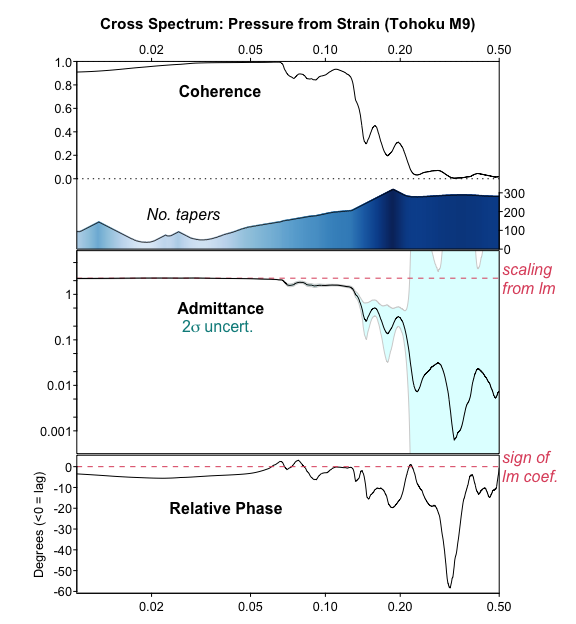All of this functionality is available in the function cross_spectrum.

1. previously known as the Plate Boundary Observatory↩︎

2. Relative changes in borehole diameter, which can be related to volume strain in the rock↩︎